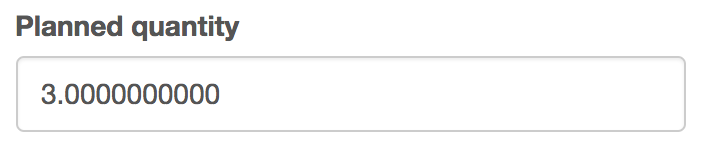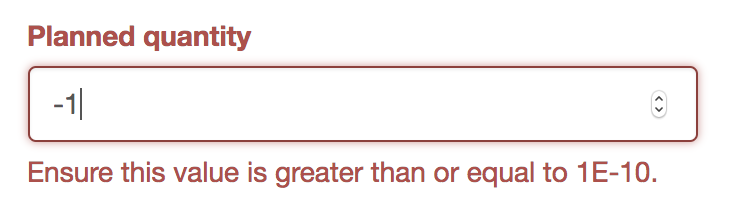Categories

# Wrangling with Django’s DecimalField and representations

I’ve been building an application that needs precicion as it is dealing with money, finance and trading. For these tasks there is a special datatype called decimal.Decimal that python has built in. It has many advantages but has a few disadvantages when displaying the decimal to the user.

1. The trailing zeroes are shown (which is unnatural to the normal person)
2. Sometimes python decides to show the simplist form in scientific notation

let’s get into it…

### Python’s Decimal

Working with Decimal is quite nice. They are intialised with string representations of numbers and can be cast to str:


In : from decimal import Decimal

In : precise_number = Decimal('54.899721')

In : precise_number
Out: Decimal('54.899721')

In : str(precise_number)
Out: '54.899721'


Which all seems normal but maybe this won’t:


In : zeroed_num = Decimal('54.000000')

In : zeroed_num
Out: Decimal('54.000000')

In : str(zeroed_num)
Out: '54.000000'


More on this later…

### Django’s Decimal Field

Django’s Decimal Field (model field) is slightly different as now we are integrating the saving of this python decimal in a database. That is why we need to set max_digits and decimal_places. So you need to think about the maximum precision expected and maximum total digits in a decimal stored you will be working with in your system. No need to go overboard and from my experience this field can be modified to go bigger.


planned_quantity = models.DecimalField(
max_digits=19, decimal_places=10,
blank=True, null=True
)


So with the above example I can store 999,999,999.1010101010 but nothing more precise or with more digits.

Everything feels great.

### Trailing Zeroes

Now after creating a record and now returning to edit the record the form will output the number including the trailing zeroes:Which just feels wrong. It should just show 3 like a normal human would expect. We saw this a bit earlier and I did a bit of digging. After searching django remove trailing zeroes the result mentioned normalize() which is a function of Decimal:


In : str(zeroed_num)
Out: '54.000000'

In : str(zeroed_num.normalize())
Out: '54'

In : zeroed_num.normalize()
Out: Decimal('54')


As mentioned in the normalize docs it doesn’t just strip the trailing zeroes it also converts 0 to scientific notation.

So it will exhibit this behaviour:


In : damn_decimal = Decimal('600')

In : damn_decimal
Out: Decimal('600')

In : damn_decimal.normalize()
Out: Decimal('6E+2')

In : str(damn_decimal.normalize())
Out: '6E+2'


So normalize is not what we want.

### Scientific Notation

So as we alluded to previously sometimes things will just look wierd to the non-scientific on our website:This error message is created by the validator:


planned_quantity = models.DecimalField(
max_digits=19, decimal_places=10,
blank=True, null=True,
validators=[MinValueValidator(Decimal('0.0000000001'))]
)


So things are looking a bit tricky and difficult to work with. Even more so when you bring in validation messages and positive decimal validation.

For now though let us just try and display decimals nicely to the user

## The Fix

So we know that normalize is not going to cut it. We’ll need a bit more and that comes from format.

So the intial data of the form will need to be modified for the user and to do that when loading the form do:


for key in form.initial.keys():
if isinstance(form.initial[key], decimal.Decimal):
form.initial[key] = '{0:f}'.format(form.initial[key].normalize())


The important thing here is getting all our decimal fields and then normalizing them.

Then displaying the number in a fixed-point float format with: x = '{:f}'.format(my_dec.normalize())

So that is it. There is a better way of doing this I think and that would be modifying the model and I’m looking at that now.